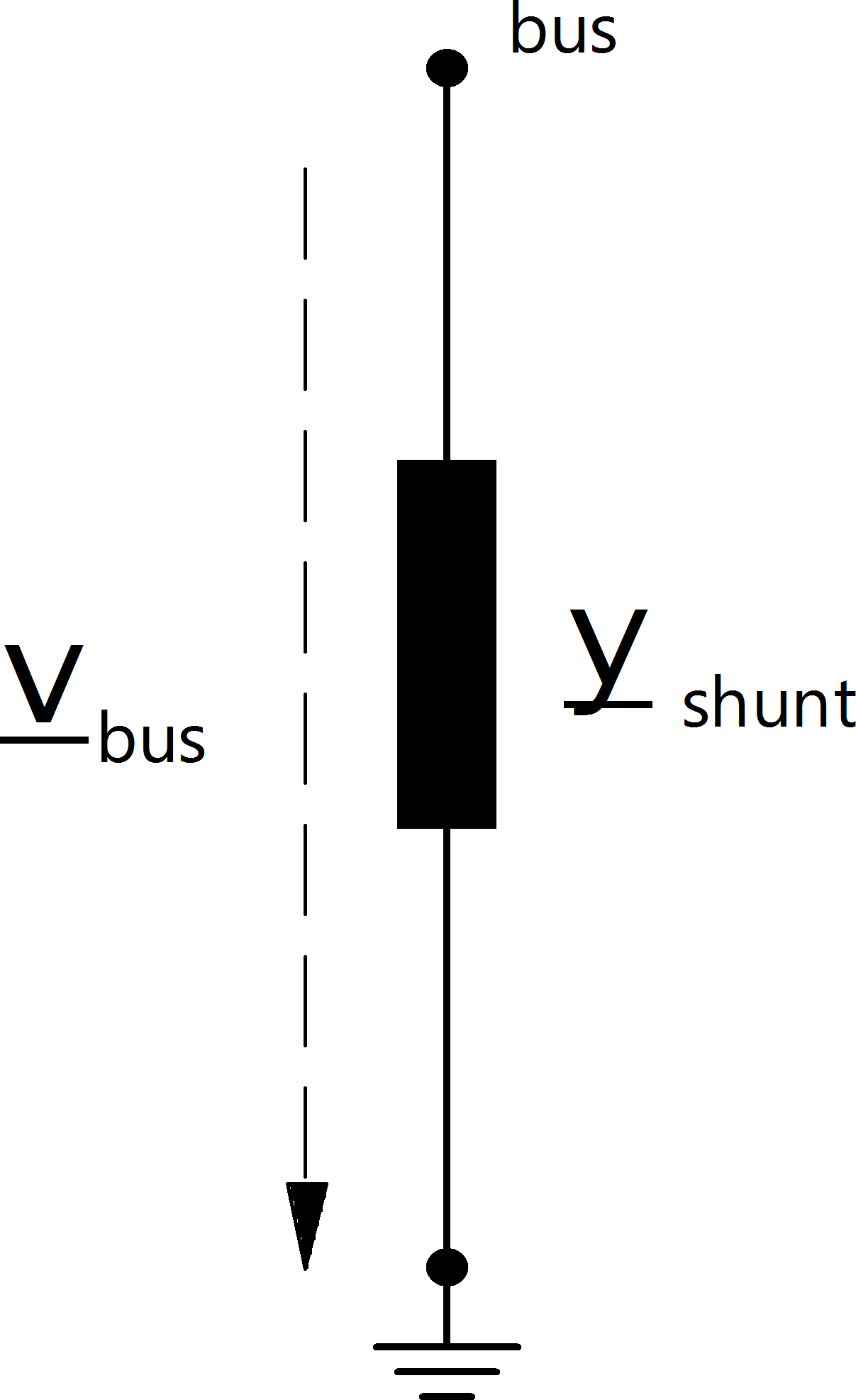# Shunt

## Create Function

pandapower.create_shunt(net, bus, q_mvar, p_mw=0.0, vn_kv=None, step=1, max_step=1, name=None, in_service=True, index=None)

Creates a shunt element

INPUT:

net (pandapowerNet) - The pandapower network in which the element is created

bus - bus number of bus to whom the shunt is connected to

p_mw - shunt active power in MW at v= 1.0 p.u.

q_mvar - shunt susceptance in MVAr at v= 1.0 p.u.

OPTIONAL:

vn_kv (float, None) - rated voltage of the shunt. Defaults to rated voltage of connected bus

step (int, 1) - step of shunt with which power values are multiplied

max_step (boolean, True) - True for in_service or False for out of service

name (str, None) - element name

in_service (boolean, True) - True for in_service or False for out of service

index (int, None) - Force a specified ID if it is available. If None, the index one higher than the highest already existing index is selected.

OUTPUT:

index (int) - The unique ID of the created shunt

EXAMPLE:

create_shunt(net, 0, 20)

pandapower.create_shunt_as_capacitor(net, bus, q_mvar, loss_factor, **kwargs)

Creates a shunt element representing a capacitor bank.

INPUT:

net (pandapowerNet) - The pandapower network in which the element is created

bus - bus number of bus to whom the shunt is connected to

q_mvar (float) - reactive power of the capacitor bank at rated voltage

loss_factor (float) - loss factor tan(delta) of the capacitor bank

OPTIONAL:

same as in create_shunt, keyword arguments are passed to the create_shunt function

OUTPUT:

index (int) - The unique ID of the created shunt

## Input Parameters

net.shunt

 Parameter Datatype Value Range Explanation name string name of the shunt bus* integer index of bus where the impedance starts p_mw* float $$\geq$$ 0 shunt active power in MW at v= 1.0 p.u. q_mvar* float shunt reactive power in kvar at v= 1.0 p.u. vn_kv* float $$>$$ 0 rated voltage of the shunt element step* integer $$\geq$$ 1 step position of the shunt in_service* boolean True / False specifies if the shunt is in service.

*necessary for executing a power flow calculation.

## Electric ModelThe power values are given at $$v = 1$$ pu and are scaled linearly with the number of steps:

\begin{align*} \underline{S}_{shunt, ref} &= (p\_mw + j \cdot q\_mvar) \cdot step \end{align*}

Since $$\underline{S}_{shunt, ref}$$ is the apparent power at the nominal voltage, we know that:

\begin{align*} \underline{Y}_{shunt} = \frac{\underline{S}_{shunt, ref}}{vn\_kv^2} \end{align*}

Converting to the per unit system results in:

\begin{align*} \underline{y}_{shunt} &= \frac{\underline{S}_{shunt, ref}}{V_{N}^2} \cdot Z_{N}\\ &= \frac{\underline{S}_{shunt, ref}}{V_{N}^2} \cdot \frac{V_{N}^2}{S_{N}} \\ &= \frac{S_{shunt, ref}}{S_{N}} \end{align*}

with the reference values for the per unit system as defined in Unit Systems and Conventions.

## Result Parameters

net.res_shunt

 Parameter Datatype Explanation p_mw float shunt active power consumption [MW] q_mvar float shunt reactive power consumption [MVAr] vm_pu float voltage magnitude at shunt bus [pu]
\begin{align*} p\_mw &= Re(\underline{v}_{bus} \cdot \underline{i}_{shunt}) \\ q\_mvar &= Im(\underline{v}_{bus} \cdot \underline{i}_{shunt}) \\ vm\_pu &= v_{bus} \end{align*}